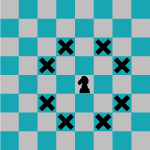# From Linear Array to Row/ColumnA chessboard is a gird, traditionally eight-by-eight squares for 64 positions. This game matrix can be implemented in code in a variety of ways, with a two-dimensional array being the most obvious. But this option isn’t what I chose when programming how a knight moves (from last week’s Lesson).

Trust me, I did a lot of trial and error.

At first I decided to eschew using a specific data structure for the chessboard. Instead, I would store the knight’s position in a structure, similar to the `position` structure from last week’s Lesson:

```struct position {     int row;     int col; };```

This structure would be filled with random values and bandied about various functions that manipulate the knight and plot where it can move. I wrote a lot of code around this approach — but it was clumsy and I grew annoyed and unhappy.

A two-dimensional array seems obvious, but it also adds some complexity I didn’t want to deal with. After all, a 2D array in C is really a one-dimensional array all gussied up. Even so, I abandoned both the structure and 2D array approaches and instead use a single integer value to represent the knight’s position based on the chessboard’s size.

To allow for a chessboard of varying size (one thing math geeks like to play with), I use the defined constant `SIZE` to set the matrix. Yet, I still need to know the row and column where a piece sits to calculate its potential moves. Therefore some translation is required.

The following code plows through all 64 (or `SIZE*SIZE`) possible locations on a chessboard, using two statements that report each square’s row and column position for the given grid size.

### 2022_11_05-Lesson-a.c

```#include <stdio.h>

#define SIZE 8

int main()
{
int square,row,column;

for( square=0; square<SIZE*SIZE; square++ )
{
/* calculate row and column */
column = square % SIZE;
row = (square-column)/SIZE;
printf("%d:%d ",row,column);
if( column==SIZE-1 )
putchar('\n');
}

return(0);
}```

The for loop processes each of the “squares” in the matrix. based on values from 0 through the matrix size (`SIZE*SIZE`). Line 12 calculates the column first, which is the square‘s value modulo `SIZE`. This value helps Line 13 find the row number: subtracting the column offset from the square value, then dividing by constant `SIZE`.

Line 15 adds a newline after each row of grid pairs is output:

```0:0 0:1 0:2 0:3 0:4 0:5 0:6 0:7 1:0 1:1 1:2 1:3 1:4 1:5 1:6 1:7 2:0 2:1 2:2 2:3 2:4 2:5 2:6 2:7 3:0 3:1 3:2 3:3 3:4 3:5 3:6 3:7 4:0 4:1 4:2 4:3 4:4 4:5 4:6 4:7 5:0 5:1 5:2 5:3 5:4 5:5 5:6 5:7 6:0 6:1 6:2 6:3 6:4 6:5 6:6 6:7 7:0 7:1 7:2 7:3 7:4 7:5 7:6 7:7```

This output represents the chessboard matrix as a series of row:column pairs, successfully translating sequential values zero through 63. The code confirms that I’m able to extract row/column pairs based on linear values.

My next task in building a program to plot how a knight moves is to set a virtual knight on the chessboard at a random position and report the position.

### 2022_11_05-Lesson-b.c

```#include <stdio.h>
#include <stdlib.h>
#include <time.h>

#define SIZE 8

int main()
{
int knight,row,column;

/* seed the randomizer */
srand( (unsigned)time(NULL) );

/* pick a random square */
knight = rand() % (SIZE*SIZE);
printf("The knight is at square %d\n",knight);

/* calculate row and column */
column = knight % SIZE;
row = (knight-column)/SIZE;
printf("That's row %d, column %d\n",row,column);

return(0);
}```

Position for variable knight is assigned at Line 15, a random value from zero to 63 inclusive. This value is reported (output) at Line 16. Then Lines 19 and 20 extract the row and column values. Here’s a sample run:

```The knight is at square 47 That's row 5, column 7```

This code represents the typical testing I do when I attempt a complex project like calculating where a knight can move on a chessboard.

The next step is to combine the solution from this month’s Exercise, so that the knight’s position is output in the color-coded chessboard on the text screen. This task is covered in next week’s Lesson.